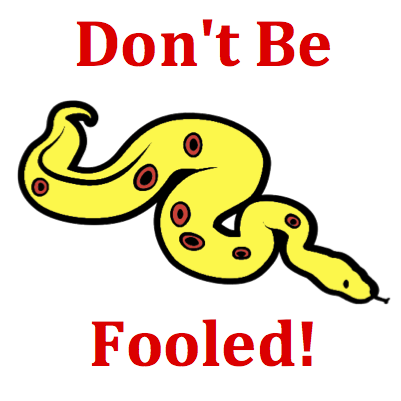# Kinematic Graphing - Mission KG7 Detailed HelpThe motion of six objects - A, B, C, D, E, and F - are represented by lines on a velocity-time graph. Write the letter(s) for any object which has a constant velocity (for the entire motion). List all that apply ... .Velocity-Time Graphs: Velocity versus time graphs represent changes that occur in an object's velocity with respect to time. The slope of the line is the acceleration (change in velocity divided by the change in time) of the object. The area under the line (between the line on the graph and the time axis) is the displacement of the object.It is not unusual for a student to confuse position-time graphs with velocity-time graphs. They know that moving with a constant velocity is represented on a position-time graph by a straight diagonal line. So they instantly begin looking for the same line on this graph. But don't be fooled! This is a velocity-time graph and the slope is the acceleration. A diagonal line with a constant slope on a velocity-time graph is a line with a constant acceleration (meaning that there is a velocity change).This is an example of a question that can be answered with a mixture of good math sense and logical thinking. The graph is a plot of velocity. Any motion that is characterized by a constant velocity would be represented by a line whose velocity coordinate is staying at the same level. Such a line is a horizontal line. A constant velocity motion is represented on a velocity-time graph by a horizontal line.